# Switched Filter Phase Shifters

This phase shifter bit is related to the lumped-element high-pass/low-pass bit, but doesn't require back-to-back switches so it has less loss. Like most phase shifters, it is a reciprocal, passive network. It provides flat phase shift across a moderate bandwidth (>10%), unlike a switched line phase shifter. In practice the switched filter phase shifter is useful for bits up to 90 degrees phase shift, but 180 bits can be constructed by cascading two switched filter 90 degree bits. This style of phase bit can be made to work at millimeterwave frequencies.

### Switched filter phase shifter patents

There are many, many examples of switched filter phase bits. You can find many of them have been patented. The distinction between this form of bit and others gets blurry after a while. Here's a few patents to read:

4,733,203

Oops, that one is really a high-pass/low-pass style! More to come.

## Switched high-pass filter phase bit

References for this concept appear at the bottom of the page. You can see an excellent example of this phase bit here.

This type of filter is extremely versatile, and is used in many phase shifters. It offers moderate bandwidth, perhaps 30%, and phase shift from 11 to 90 bits have been made on compound semis as well as CMOS and BiCMOS SiGe. It can be simplified for an 11 degree bit (more on that later), and 180 bits can be realized by cascading two 90s of this style. The first two bits on the left of this picture use this network, for 22 and 11 degrees.

Note that when we say "22" we mean 22.5, and "11" means 11.25 degrees, so don't bother pointing this out to us. But if you think you can actually design a phase shifter bit with 0.25 degrees of accuracy, we'd like to place a small wager with you on your next effort...

Shown below is the simplified block diagram of how it works. On the left is the "bypass" state, where a pi-network filter's response is corrupted by a pair of switches. SW1 shorts out the series capacitor, SW2 disconnects the shunt inductors L1 from ground. It is important to note that SW2 provides a single node to the shunt inductors, such that they are connected in series in this state. You might ask "what's the difference" between this and two switches to ground acting independently. There's a MAJOR difference. In microwaves, there's really no such thing as a perfect lumped element, in practice the inductors will be transmission lines with capacitance to ground. If you left them hanging open, they would form open circuit stubs and detune the circuit! In the high-pass state, SW1 opens and SW2 shorts, and the high-pass pi filter is realized. The filter's values are chosen such that is has almost effect on amplitude in the band, but is provides the required transmission phase.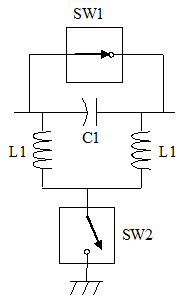Bypass state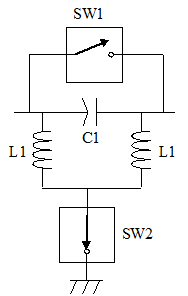High-pass state

By the way, you'll find similar schematics in a Word document you can get for free in our download area, it's called Electronic_Symbols_xx.doc, where xx is the revision number.

Our definition of phase states is such that the more positive phase state is the reference state. Therefore the high-pass state is the reference state, and the phase state is the bypass state (phase shift is negative in value). Don't be too confused by this, it is merely our convention!

Let's take a look at how such a phase shifter is actually implemented in a MMIC. Switch FETs are well known for their versatility in control circuits, they behave like a resistors when on, and a capacitor when off. That "parasitic" capacitor can be employed in this style phase bit to provide not just SW1, but also the capacitor that is required, in some cases. But the capacitance can work against you, especially at millimeterwave frequencies), you need to resonate it with a parallel inductor to get a clean ON/OFF switch for SW2.

#### Goldilocks and the three FETs...

In the left figure below, the FET has been sized to provide the exact capacitance needed in the HPF. This is not always possible, convenient or wise to do this. It's often a coward's way out to design a phase shifter bit with a single sized FET that has previously measured S-parameters or a verified equivalent circuit model, rather than scale a model and take your chances. In the other two pictures, a separate C1 capacitor is used to increase the series capacitance of the HPF, or decrease it.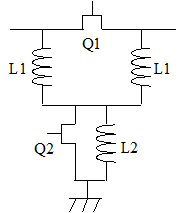FET Q1 sized exactlyFET Q1 too small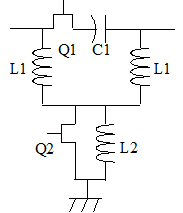FET Q1 too large

Goldilocks would be proud of you if you sized Q1 just right! Notice L2 is used to resonate the off-capacitance of FET Q2 to a near-open circuit.

Here's the equations for C1 and L1:

C1=1/(Z0*2*pi*F*sin(phase_shift)

L1=Z0/(2*pi*F*tan(phase_shift/2)

Note that these are the same equations used in the high-pass/low-pass phase shifter's high-pass pi arm. You don't have to be named Einstein to have guessed that! By the way, there's several ways to express the tangent of a half-angle (which is used in the L1 formula.) Check it out on Wikipedia.

Here's the values for L1 and C1 for four phase bits, at 10 GHz:

 Frequency (GHz) Phase shift (degrees) L1 (nH) C1 (pF) 10 11.25 8.080 1.632 10 22.5 4.001 0.832 10 45 1.921 0.450 10 90 0.796 0.318

#### ADS model of ideal switched HP filter phase bit

Now let's look at the predicted response for our ideal switched high-pass filter using ADS. The schematic below shows a two circuits, one is the reference state, one is the phase shift state. The parameter "ON" is what throws the state. Are we brilliant, or what? No need to answer, that's a rhetorical question. The desired phase shift and center frequency are entered here. We chose 10 GHz for center frequency.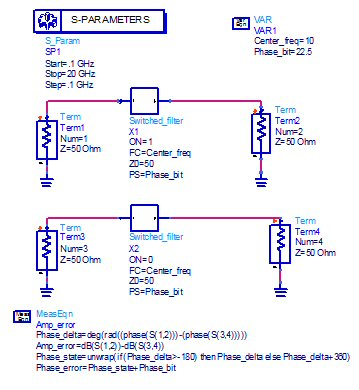Clicking into the "Switched_filter" schematic, we see the equations for C1 and L1 are entered. Also, R1 and R2 are merely on/off elements that are used to drive the schematic between two states. When parameter "ON" is set to 1, R1 goes to nearly short circuit, and R2 goes to nearly open circuit.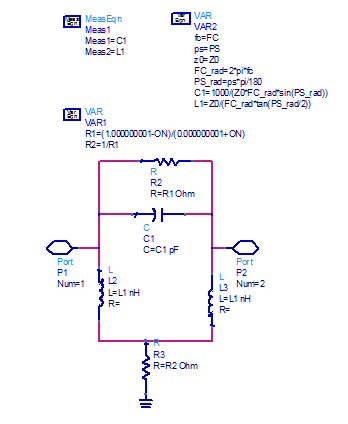Now let's look at the phase shift and magnitude of transmission and reflection coefficients of the phase shift state. The reference state is trivial, it has perfect S-parameters which need not be plotted.

Below is the 11 degree bit response. Note that phase shift is plotted over 40% bandwidth, while the magnitudes are plotted over a wider band so you can observe the HP characteristic. The phase shift has a tilt to it, an ideal phase bit would be completely flat. Fear not, by the time this design is fully developed, the reference state will have low-pass response just due to interconnects, and the phase shift can be flattened out over a respectable bandwidth, like a high-pass/low-pass phase bit. The transmission/reflection coefficients show a textbook response of a N=3 high-pass filter, with 10 GHz way above the corner frequency. Keep an eye on the corner frequency as we increase the phase shift of the design...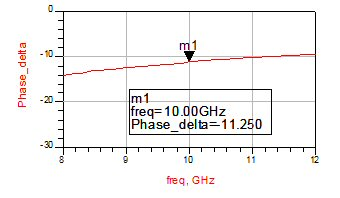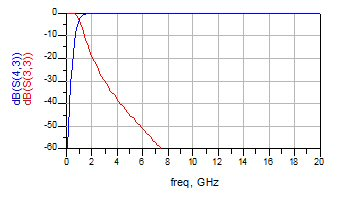Phase shift Reflection and transmission coefficients,HP state

Here's a 22 degree bit. It has a little more phase tilt to it. Also, the corner frequency is walking toward 10 GHz.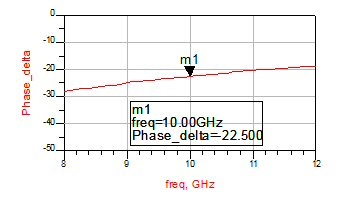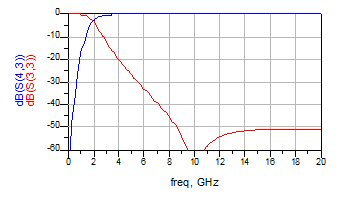Phase shift Reflection and transmission coefficients,HP state

Here's the 45 degree bit. More phase tilt, and the HP corner frequency is now around 5 GHz.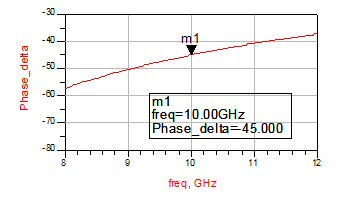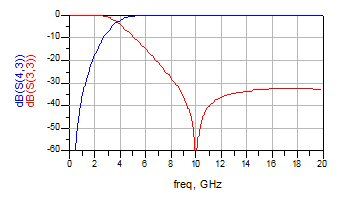Phase shift Reflection and transmission coefficients,HP state

Here's the 90 degree bit. Still more tilt, and the corner frequency is getting very close to 10 GHz.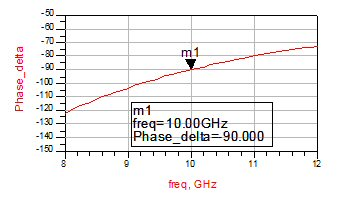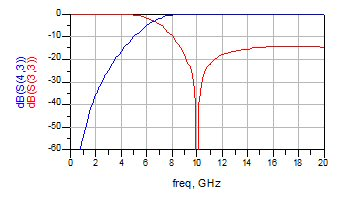Phase shift Reflection and transmission coefficients,HP state

What about a 180 degree bit? Turns out you can't make one this way! Try it if you're skeptical... Let's look at a mythical 170 degree bit (you'd never need one) using the switched HP filter. Yes, we have produced a 170 degree phase shift at 10 GHz, but the bandwidth stinks!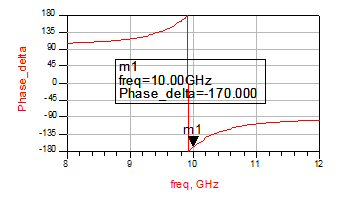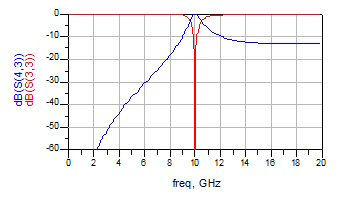Phase shift Reflection and transmission coefficients,HP state

It's time for a Microwaves101 Rule of Thumb: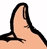Switched filter phase shifter bits, either high pass, or low pass, are not useful above 90 degrees of phase shift. For a 180 bit, you must cascade to 90s, or use an alternate structure. The preferred structure is a high-pass/low-pass bit.

### Alternate 11 degree bit

Earlier on this page we hinted that the 11 degree bit could be simplified. Never say "alluded to" when you mean "hinted at", especially if your normal speech vocabulary is limited like most engineers... it sounds like you are stretching yourself.

For the 11 degree bit, you can eliminate SW2, and ground the inductors. This is a compromise, now the reference state will have a non-ideal phase shift (also a high-pass characteristic).

In the original network, the phase shift that is achieved is split equally between the two inductors and the capacitor C1. When you tie the inductors to ground, in order to get 11.25 degrees phase shift, you need to size the capacitor for 22.5 degrees phase shift.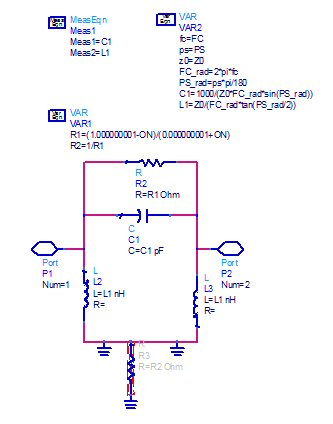Here are the phase shift between the states, as well as the reflection and transmission coefficients for both states. Because we have compromised the network, the reference state is no longer ideal. You can allocate the compromise any way you want between the two states, we chose to leave the 11 degree state ideal (it is the same as the 22 degree state above).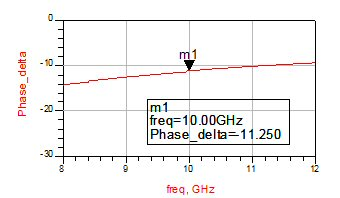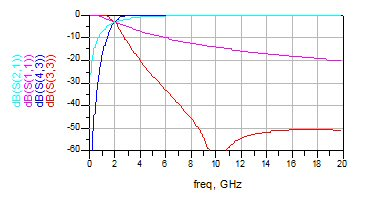Phase shift Reflection and transmission coefficients,HP state

This plot shows the phase of the transmission coefficient of both states. Here you can see the non-ideal response of the reference state.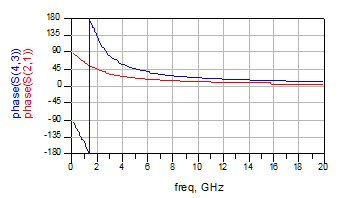### Switched high-pass filter phase shifter references

There are many references to switched filter phase shifters in the literature.

Some of the early work was done by Anthony Jacomb-Hood of General Electric (at the time).

Perhaps the most instructive reference was written by two TriQuint authors. This paper provides the equations for computing lumped element values for switched filter phase shifters (which we already showed you).

A Compact 5-bit Phase-Shifter MMIC for K-band Satellite Communication Systems
Campbell, C.F.; Brown, S.A.
IEEE Transactions on Microwave Theory and Techniques
Volume 48, Issue 12, December 2000

Other authors have published similar works, including Gabe Rebeiz's team at UCSD.

Author : Unknown Editor